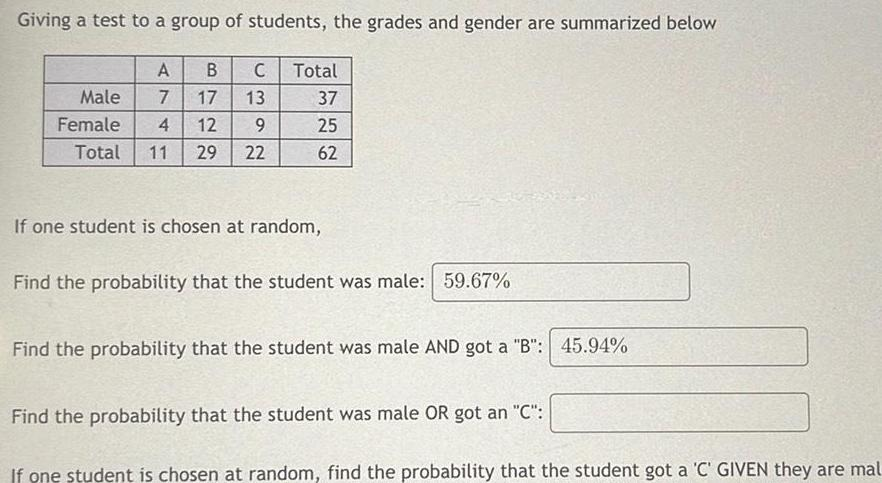Question:

# Giving a test to a group of students the grades and gender

Last updated: 11/20/2023Giving a test to a group of students the grades and gender are summarized below A C Male 7 17 13 Female 4 12 9 Total 11 29 22 B Total 37 25 62 If one student is chosen at random Find the probability that the student was male 59 67 Find the probability that the student was male AND got a B 45 94 Find the probability that the student was male OR got an C If one student is chosen at random find the probability that the student got a C GIVEN they are mal